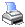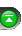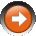NinjaScript > Language Reference > Strategy > TradeDefinition

A Trade is a completed buy/sell or sell/buy transaction. It consists of an entry and exit execution.

Example 1:
Buy 1 contract at a price of 1000 and sell 1 contract at a price of 1001 is one complete trade.

Example 2:
Buy 2 contracts at a price of 1000 and sell the 1st contract at a price of 1001, then sell the 2nd contract at a price of 1002 are two completed trades.

In the second example above, two trade objects are created to represent each individual trade. Each trade object will hold the same entry execution for two contracts since this single execution was the opening execution for both individual trades.

Methods and Properties

 Commission A double value representing the commission of the trade Entry Gets an IExecution object representing the entry Exit Gets an IExecution object representing the exit ProfitCurrency A double value representing profit in currency for one traded unit. Multiply this value by the trade Quantity to get the total profit in currency for the trade. ProfitPercent A double value representing profit as a percentage ProfitPoints A double value representing profit in points for one traded unit. Multiply this value by the trade Quantity to get the total profit in points for the trade. Quantity An int value representing the quantity of the trade ToString() A string representation of the Trade object

Examples